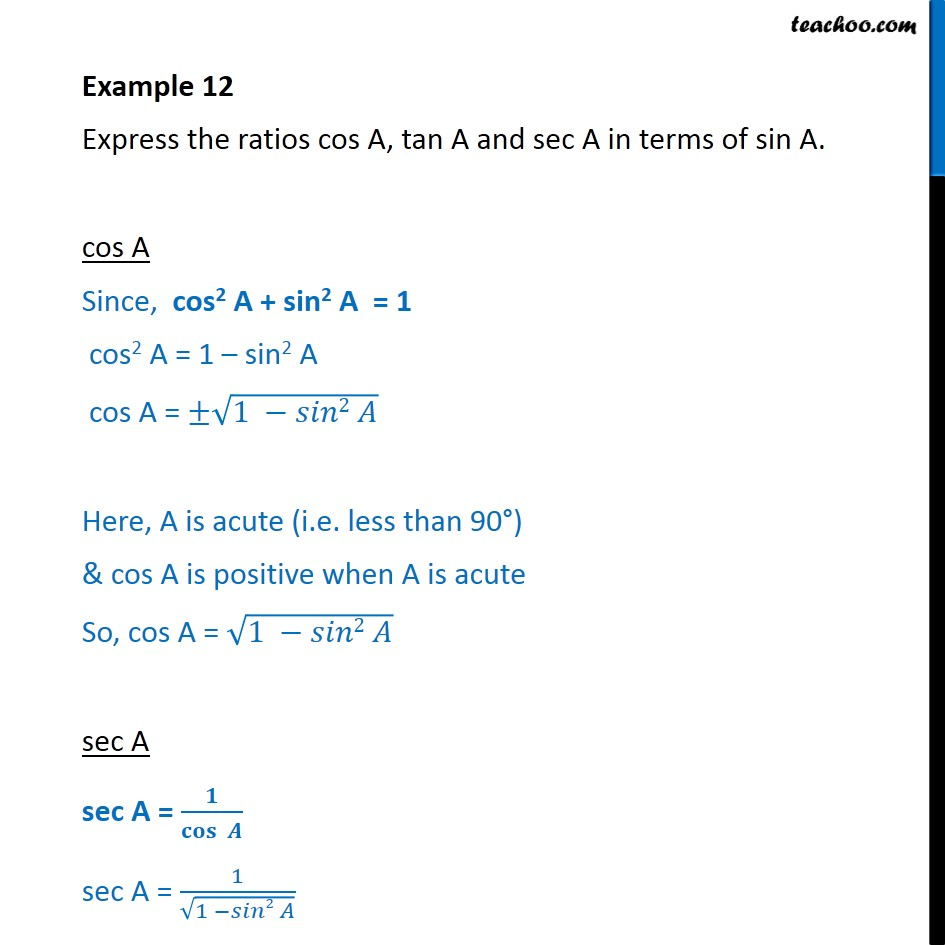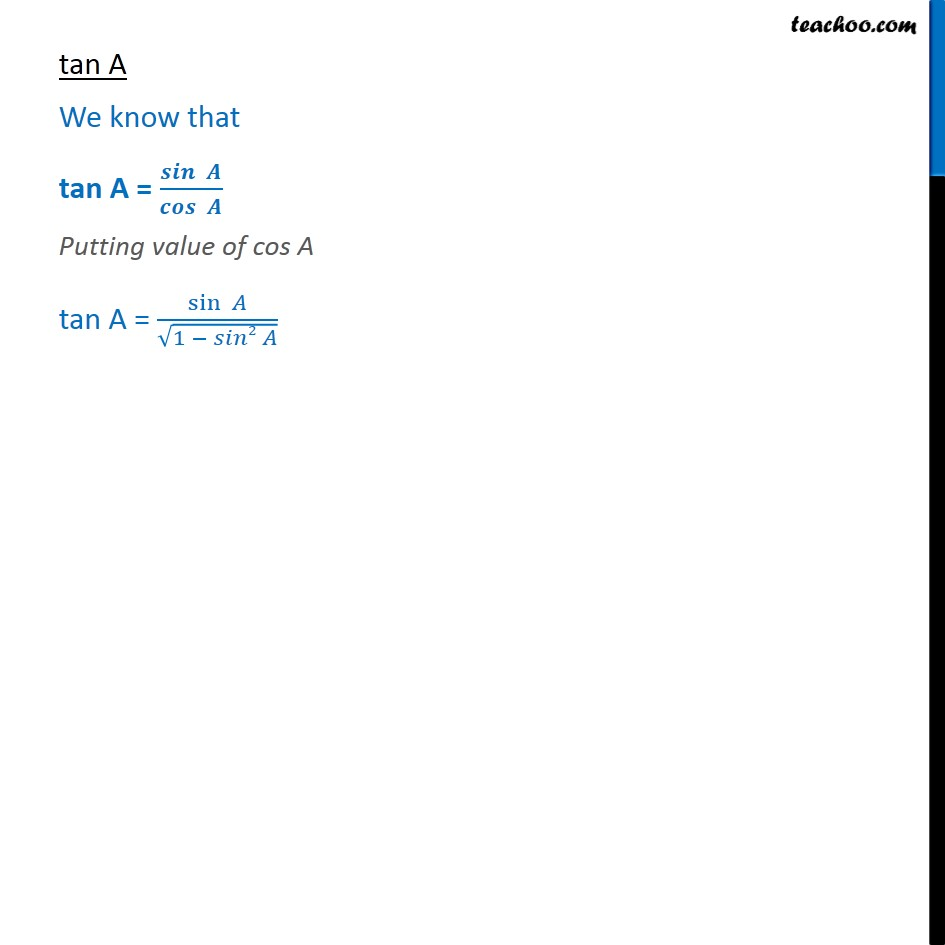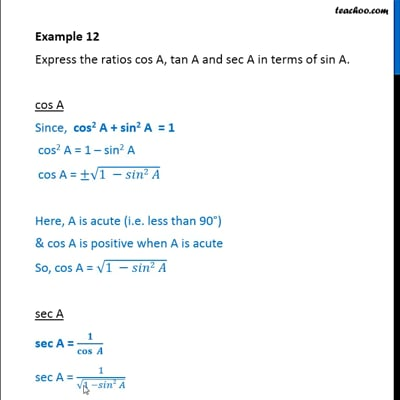Examples

Chapter 8 Class 10 Introduction to Trignometry
Serial order wiseThis video is only available for Teachoo black users

Maths Crash Course - Live lectures + all videos + Real time Doubt solving!

### Transcript

Example 12 Express the ratios cos A, tan A and sec A in terms of sin A. cos A Since, cos2 A + sin2 A = 1 cos2 A = 1 – sin2 A cos A = ±√(1 −𝑠𝑖𝑛2 𝐴) Here, A is acute (i.e. less than 90°) & cos A is positive when A is acute So, cos A = √(1 −𝑠𝑖𝑛2 𝐴) sec A sec A = 𝟏/𝐜𝐨𝐬⁡〖 𝑨〗 sec A = 1/√(1 −𝑠𝑖𝑛2 𝐴) tan A We know that tan A = 𝒔𝒊𝒏⁡〖 𝑨〗/𝒄𝒐𝒔⁡〖 𝑨〗 Putting value of cos A tan A = sin⁡〖 𝐴〗/√(1 − 𝑠𝑖𝑛2 𝐴)